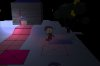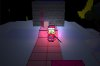#### Gun_Goose

##### Member
Hi, I am trying to implement a 3D fog of war shader (i have been struggling with this for a while). I have tried to build one from scratch as well as implement on I got off the market place and each time, I have run into a similar problem. I am able to create the proper effect, however the the light is not center on the position of the character I pass it (it even follows the character, expect seems to move a different ). I think it might be a problem with the fact that I am drawing everything with a matrix, and the positions would not line up, but I am not sure anymore. Any ideas?The current shader I am testing is one I found on the market place:
vertex:

Code:
``````//
//
attribute vec4 in_Position;
//attribute vec3 in_Normal;
attribute vec4 in_Colour;
attribute vec2 in_TextureCoord;
varying vec2 v_vTexcoord;
varying vec4 v_vColour;
varying vec4 WorldPos;
void main()
{
gl_Position = gm_Matrices[MATRIX_WORLD_VIEW_PROJECTION] * in_Position;
WorldPos = gm_Matrices[MATRIX_WORLD] * in_Position;
v_vColour = in_Colour;
v_vTexcoord = in_TextureCoord;
}``````
Fragment:
Code:
``````//
//
varying vec2 v_vTexcoord;
varying vec4 v_vColour;
varying vec4 WorldPos;
uniform vec3 u_fog_colour; //Red, Green, Blue
uniform vec3 u_fog_height; //Z near, Z height, Falloff
uniform vec3 u_camera_position; //Cameras X, Y, Z
void main()
{
vec4 base = texture2D(gm_BaseTexture, v_vTexcoord);

float dist = length(WorldPos.xyz - u_camera_position);
float fog = u_fog_height.z * smoothstep(u_fog_colour.z, u_fog_height.x, u_fog_height.y - WorldPos.z);

float ext = exp2(-dist * fog);
vec3 finalColor = base.rgb * vec3(ext,ext,ext) + u_fog_colour.rgb * (1.0 - ext);

gl_FragColor = /*v_vColour * */vec4(finalColor, 1.0);
}``````
Here is also how I am calling the shader in the post draw event:
Code:
``````shader_set(sh_fog_try);

draw_surface(application_surface,0,0)
p_x, p_y, p_z are the characters coordinate. I have also tried switching them for the coordinates of my camera object but ended up with the same result.

#### flyingsaucerinvasion

##### Member
I notice sometihng strange. You've got a vec4 in_Position attribute. Usually they are vec3. Have you wrote a w component to your vertex position attributes in your vertex buffer? If so, it must have a value of 1 to work with this shader. But why does the in_Position attribute have 4 components in the first place?

The arguments in the smoothstep function don't make sense to me.

Did you pay money for that shader?

#### Gun_Goose

##### Member
I did not pay money for the shader, it was free on the asset store. I was using it to test to see if the problem was that I was passing it coordinates wrong. I have a simpler shader that I better understand which is supposed to accomplish a similar foggy effect. (i think it might be easier to troubleshoot) (I posted it below). It has a similar problem where it is off center.However, after playing with it for a while. I think the problem might be with the fact I am using a surface and that it would be utilizing GUI coordinates. For instance, when currently drawing it like:

Code:
``````shader_set(sh_spooky)

draw_surface(application_surface,0,0)

in the post draw event after disabling application surface in the create event. However, when I change p_x and p_y to display_get_gui_width()/2 and display_get_gui_height()/2 respectively, the shader looks like:It looks much closer to how I want it. Although I am not sure how I would change 3d coordinates into GUI coordinates. Aren't GUI coordinates 2d?

Vertex:

Code:
``````//
//
attribute vec3 in_Position;                  // (x,y,z)
//attribute vec3 in_Normal;                  // (x,y,z)     unused in this shader.
attribute vec4 in_Colour;                    // (r,g,b,a)
attribute vec2 in_TextureCoord;              // (u,v)

varying vec2 v_vTexcoord;
varying vec4 v_vColour;
varying vec3 v_vPosition;
varying vec3 v_vWorld;

uniform vec3 pos;

void main()
{

vec4 object_space_pos = vec4( in_Position.x, in_Position.y, in_Position.z, 1.0);
gl_Position = gm_Matrices[MATRIX_WORLD_VIEW_PROJECTION] * object_space_pos;

v_vColour = in_Colour;
v_vTexcoord = in_TextureCoord;
v_vPosition = in_Position;

v_vWorld = (gm_Matrices[MATRIX_WORLD] * object_space_pos).xyz;
}``````
Fragment:
Code:
``````//
//
varying vec2 v_vTexcoord;
varying vec4 v_vColour;
varying vec3 v_vPosition;
varying vec3 v_vWorld;

uniform vec3 pos;

void main()
{

vec4 base_col = v_vColour * texture2D(gm_BaseTexture, v_vTexcoord);
gl_FragColor = vec4(base_col.rgb, base_col.a*(100.0/(distance(pos,v_vWorld))));
}``````

#### flyingsaucerinvasion

##### Member
If you want the effect to be 3d, you'll want to apply the shader as you draw everything in your world.